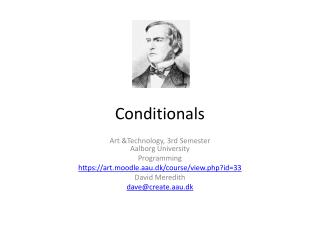# Conditionals - PowerPoint PPT PresentationDownload PresentationConditionals

ConditionalsDownload Presentation## Conditionals

- - - - - - - - - - - - - - - - - - - - - - - - - - - E N D - - - - - - - - - - - - - - - - - - - - - - - - - - -
##### Presentation Transcript

1. Conditionals Art &Technology, 3rd SemesterAalborg University Programming https://art.moodle.aau.dk/course/view.php?id=33 David Meredith dave@create.aau.dk

2. Overview • Boolean expressions • Conditional expressions: “if”, “else”, “else if” • Logical operators: “&&” and “||” • Multiple rollovers • Boolean variables • Bouncing ball

3. Boolean expressions • Expressions in normal language can be true, false, ambiguous or meaningless, e.g. • TRUE: “The picture shows George Boole (1815-1864), an English mathematician who invented formal logic” • FALSE: “The picture shows Mickey Mouse” • MEANINGLESS: “Flurby zilches blab wibberously” • AMBIGUOUS: “Slow children at play”

4. Boolean expressions • A boolean expression is one that has a truth value • This truth value can be either false or true • A boolean expression is usually a statement about the relationship between two numbers or variables that contain numbers, e.g. 12 > 10 (true) 5 <= 10 (true) x < 5 (don’t know – depends on value of x)

5. Relational operators • In order to compare numbers in a boolean expression, we use the following operators > greater than < less than >= greater than or equal to <= less than or equal to ==equal to NB:compare with ‘=‘ which means “becomes equal to” != not equal to

6. Examples of boolean expressions • Comparing numbers 5 < 5 false 5 <= 5 true 5 = 5 error – ‘=‘ is the assignment operator and means “becomes equal to” 5 == 5 true • Comparing variables – what are the values of b and c in the following? intx = 5;inty = 7;booleanb = (x == y);booleanc = (x < y); b is false, c is true

7. if • If expression has format:if (boolean expression) {doThis();doThat();} • doThis() and doThat() are only done if boolean expression is true

8. if-else • When does it draw a green circle? • Format of If-Else is: if (bool exp) { doStuff1();} else { doStuff2();} • Does doStuff1() if bool exp is true, otherwise it does doStuff2()

9. if-else if • if-else if statement has format: if (condition1) { doStuff1();} else if (condition2) { doStuff2();} else if (condition3) { doStuff3();}…} else {doStuffOtherwise();}

10. Logical operators • We can deal with single boolean expressions:if (I have a fever) {take me to the doctor;} • But what aboutif (I have a fever OR I have a rash) { take me to the doctor;} • orif (I am stung by a bee AND I have an allergy) { take me to the doctor;} • orif (I am stung by a bee AND I am NOT breathing) { call an ambulance;} • For these, we need to be able to combineboolean expressions using the logicaloperators, “OR”, “AND” and “NOT”

11. &&, || and ! • In processing, && means logical AND || means logical OR ! means logical NOT

12. Boolean variables – programming a switch

13. Bouncing ball • Speed has two components, x and y • The “speed” is actually how much you change the coordinate value between two consecutive frames • When the ball hits an edge, the speed is reversed by negating the xSpeed if it hits a vertical edge and negating the ySpeed if it hits a horizontal edge